Next: Implementation Up: Pellet method Previous: Pellet method   Contents

Mathematical background

Let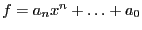be a polynomial and let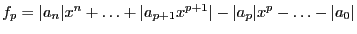be the associated polynomial. If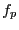has two positive roots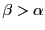, then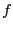has exactly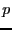roots in the disk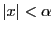and no roots in the domain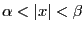.

Now assume that we have calculated the coefficients of the polynomial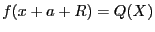where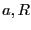are known quantities. If we determine a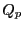polynomial that has 2 positive roots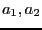in the range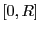, then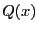has roots in the disk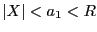. Hence the absolute value of the real part of the roots is bounded by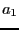. As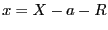we get that the real part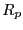of the root satisfies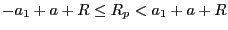. Asthis shows thathas roots whose real part is greater than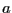.

Jean-Pierre Merlet 2012-12-20